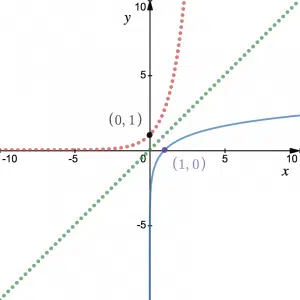# Exponentials and Logarithms in A-Level Maths

## Exponentials & Logarithms

We study Exponentials & Logarithms at A-Level Maths after having first seen exponential graphs during GCSE Maths (at the Higher level).  At GCSE, we look at the graphs of powers of integers. However, at A-Level, we look at powers of a special number $e=2.7182818284…$. The reason we study this number in particular, is because there are some simple interesting properties when we differentiate. We also look at logarithms, their graphs and equations and how they are the inverses to exponential functions.Exponential & Log graphs, Log Equations, Growth & Decay and the Differentiation of Log Functions are covered in the first year of A-Level Maths. Not a lot more is covered in the second year except to include the exponential curve in combined transformations.